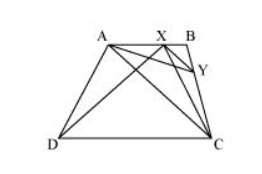# ABCD is a trapezium with AB || DC.Question. $A B C D$ is a trapezium with $A B \| D C$. A line parallel to $A C$ intersects $A B$ at $X$ and $B C$ at $Y$. Prove that ar $(A D X)=\operatorname{ar}(A C Y)$.

Solution:It can be observed that $\triangle A D X$ and $\triangle A C X$ lie on the same base $A X$ and are between the same parallels $A B$ and $D C$.

$\therefore$ Area $(\triangle \mathrm{ADX})=$ Area $(\triangle \mathrm{ACX}) \ldots(1)$

$\triangle \mathrm{ACY}$ and $\triangle \mathrm{ACX}$ lie on the same base $\mathrm{AC}$ and are between the same parallels $\mathrm{AC}$ and $\mathrm{XY}$.

$\therefore$ Area $(\triangle A C Y)=$ Area $(A C X) \ldots(2)$

From equations (1) and (2), we obtain

$\operatorname{Area}(\triangle \mathrm{ADX})=\operatorname{Area}(\triangle \mathrm{ACY})$